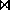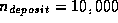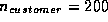Next: Simple Iteration Up: Query Processing Previous: Estimation of Access Costs

Join Strategies

1. We've seen how to estimate the size of a join. Now we look at estimating the cost of processing a join.
2. Several factors influence the selection of an optimal strategy:
• Physical order of tuples in a relation.
• Presence of indices and type of index (clustering or not).
• Cost of computing a temporary index for the sole purpose of processing one query.
3. We'll look at computing the expression depositcustomer assuming no indices exist. We also let(number of deposit tuples)(number of customer tuples)

Osmar Zaiane
Sun Jul 26 17:45:14 PDT 1998